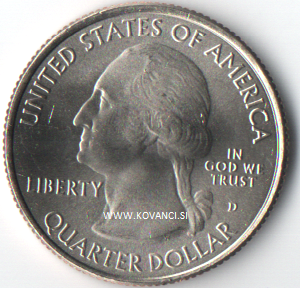!
!!

Weight: kg
New - UNC coin.

Weight: kg
New - UNC coin.

Weight: kg
New - UNC coin.

Weight: kg
New - UNC coin.

Weight: kg
New - UNC coin.

Weight: kg
New - UNC coin.

Weight: kg
New - UNC coin.

Weight: kg
New - UNC coin.

Weight: kg
New - UNC coin.

Weight: kg
New - UNC coin.

Weight: kg
New - UNC coin.

Weight: kg
New - UNC coin.

Weight: kg
New - UNC coin.

Weight: kg
New - UNC coin.

Weight: kg
New - UNC coin.

Weight: kg
New - UNC coin.

Weight: kg
New - UNC coin.

Weight: kg
New - UNC coin.

Weight: kg
New - UNC coin.

Weight: kg
New UNC coin

Weight: kg
New UNC coin

Weight: kg
New UNC coin

Weight: kg
New - UNC coin.

Weight: kg
New - UNC coin.

Weight: kg
New - UNC coin.